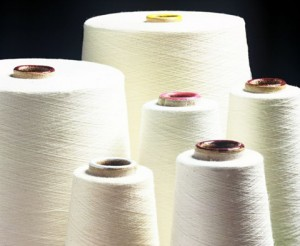# Yarn Count

## the Direct Yarn Numbering (Yarn Count) System

The count is a numerical value which is used to express the fineness or coarseness of the yarn. yarn count also indicates the relationship between the length and weight of the yarn. So, the definition of yarn count, we also can say yarn count has been introduced as a certain ratio of length to weight or weight to length.

## Types of yarn count

There two types of yarn count system. They are as follows.

1. Direct Count System
2. Indirect Count SystemTextile Yarn

## Direct Count System

The direct count is determined by the weight of a fixed length of yarn. The weight per unit length is the count (direct count). The common features of all direct yarn count systems are the length of yarn is fixed and the weight of yarn varies according to its fineness.

## We should remember in direct yarn count system

• Indirect count system Length is fixed.
• Higher the yarn number coarser the yarn and
• Lower yarn number finer the yarn.

The following formula is used to calculate the direct yarn count

N = (W × l)/L

Here,

N = Yarn count or numbering system

W = Weight of the sample

L = Length of the sample

l = Unit of the length of the sample.

## Some direct yarn count system

1. Tex system
2. Denier system
3. Decitex
4. Militex
5. Kilotex
6. Jute count

A brief definition of the above direct count system

As I have given the definition above, now here I am going to present a brief definition of the above direct count system.

Tex system: Number of grams per 1000 meters.

Denier: Number of grams per 9000 meters.

Decitex: Number of grams per 10,000 meters

Militex: Number of milligrams per 1000 meters

Kilotex: Number of kilograms per 1000 meters

Jute count: No. of pound (lb) per 14,400 yards

Indirect Count System: (Will discuss in my next article. Remain with us.)

Discussion

Example :

Now I am going to discuss direct yarn count system with an example so that we can understand it more clearly.

• Suppose 35 Tex,

What does 35 Tex define?

35 Tex means 1000 meters of yarn weighs 35 grams. If 1000 meter long yarn gives 35-gram weight then it is called 35 Tex. Hope it is clear now.

Others direct yarn count system also define so,

Wish it was helpful. Now I am going to ask you some questions about the direct count system. Try to solve these questions by yourself. Or, you can also give your answer as a comment below the post. I will discuss these following questions in my upcoming post. If you have any other questions never hesitate to ask me.

Questions :

1. 20 Tex defines…………………
2. 35 Denier defines………………..
3. 35 Tex defines………………………
4. 10 Decitex define…………………….
5. 5 Kilotex defines………………………..
6. 10 Jute count defines……………………..

Written by

Engineer Sheikh Nurja

B.Sc engineer of Textile

Yarn Count

## 3 thoughts on “Yarn Count”

1.Mazharul Islam says:

Nice article.

2.Deep says:

Very informative indeed…..

3.joyce says:

Great blog. I will definitely regularly visit here.

Scroll to top